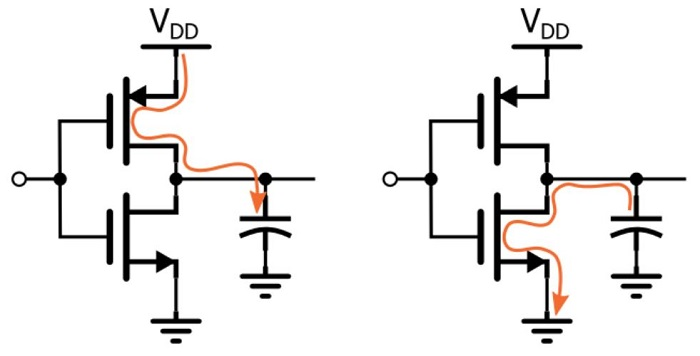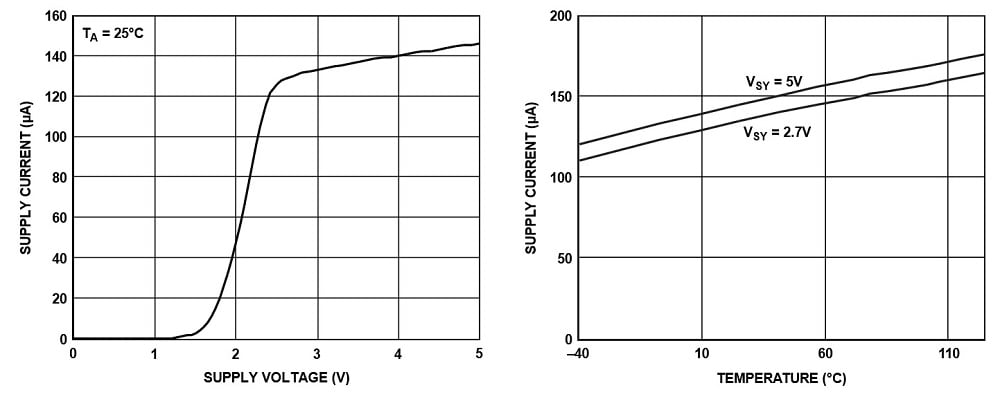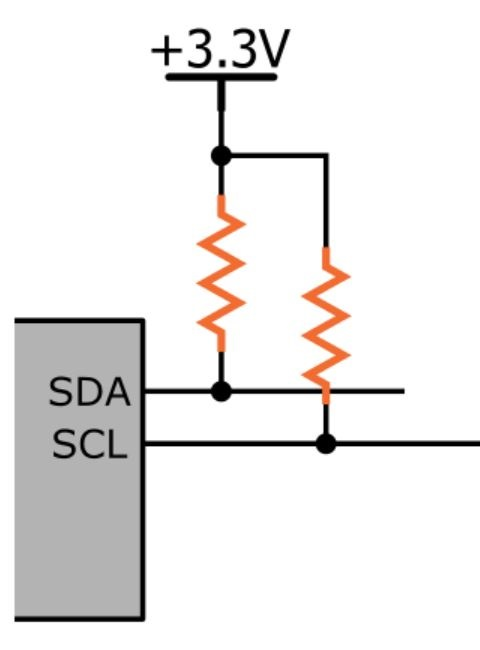Technical Article

# Introduction to Low-Power Embedded Design

February 17, 2017 by Robert Keim

## Low power consumption has become an important design goal in many electronic systems. This article introduces essential concepts and techniques.

Low power consumption has become an important design goal in many electronic systems. This article introduces essential concepts and techniques.

In this article, we’ll explore some foundational information related to minimizing power consumption in microcontroller-based embedded systems. Then, a future article will discuss specific microcontroller features and how you can use them to extend your battery life.

As implied by the previous sentence, the relentless pursuit of reduced power consumption is bound to the inevitable limitations of the battery. There certainly is nothing wrong with increasing the energy efficiency of a circuit that is powered from a wall outlet, but it’s hard to get motivated when you know that the power required by a small electronic device is so small compared to that of appliances or extensive indoor lighting.

When a product is designed primarily for battery power, everything changes. Small batteries store very limited amounts of energy, yet consumers want high-performance (read “high-power”) devices that are very compact (read “too small for a good-sized battery”) and that don’t need to be recharged every twenty minutes. What’s a designer to do?

I’m sure that battery engineers are working very hard to help us out here, but that doesn’t change the fact that we are expected to make the best of whatever energy-storage technology is currently available. This means that the primary—and, in general, the only—way to greatly extend battery life is to design circuits that “do more with less,” i.e., that achieve the desired functionality while minimizing the number of electrons that must travel from one side of the battery to the other.

### First Things First

Before we go any further, we need to make sure that everyone’s on the same page regarding terminology.

• Current: This is the rate at which electric charge is flowing through a conductor (or semiconductor). The standard unit is amperes, which is defined as coulombs (a unit of charge) per second.
• Energy: This word refers to the mysterious “property” that is transferred in the process of accomplishing something (e.g., a temperature increase, physical motion, the generation of light). A battery stores chemical energy that can be converted to electrical energy for use by a circuit. The standard unit for energy is joules.
• Power: In a scientific context, power is the rate at which energy is converted from one form to another (such as from electrical energy to heat, or electrical energy to electromagnetic radiation). The unit is watts, which is defined as joules per second.

### Charge, Energy, Power, Current . . .

We typically discuss circuitry in terms of current and power, and we discuss batteries in terms of charge or energy. For example, we might say that a particular op-amp consumes 1 mA of supply current; however, this is incomplete information if the supply current depends on the supply voltage. A power spec incorporates both of these quantities, because electrical power is calculated as voltage times current.

A battery’s capacity to sustain the functionality of an electronic circuit is expressed in ampere-hours (or usually milliamp hours, abbreviated mAh). Technically this is a unit of charge:

$$amperes\cdot hour=\frac{coulombs}{second}\cdot3600\ seconds=3600\ coulombs$$

Thus, if a circuit requires 1 mA (=0.001 coulombs per second) of current, a 1 mAh (=3.6 coulomb) battery can sustain that circuit for 3600 (=3.6/0.001) seconds, also known as one hour.

It’s good to recognize that mAh is not a unit of energy, though we might think of it as a general indicator of how much total electrical energy a given battery can deliver before it must be discarded or recharged.

The actual energy capacity of a battery depends on how much power can be supplied for how long, where power is calculated as the current supplied by the battery multiplied by the voltage across the battery terminals. (This is not a simple calculation because the voltage decreases as the battery is discharged.) Though the standard scientific unit for energy (and thus for energy capacity) is joules, we can express joules in forms that are more convenient and intuitive, such as mW·hours.

### Digital, Analog, and Passive

We can begin to analyze the power requirements of a given circuit by considering the ways in which different types of components contribute to the total current consumption.

#### Digital

The following well-known equation is used to predict the power dissipation of a single CMOS inverter:

$$P=fCV_{DD}^2$$

Actually, this is just the dynamic power dissipation. Historically CMOS technology ensures very low static power dissipation, but this is changing somewhat because as FETs shrink, static leakage current becomes more significant. Nevertheless, we’ll focus on dynamic power dissipation because there is not much that the board designer can do to reduce static power dissipation.##### A burst of current flows every time the input transitions from high to low or low to high.

Anyways, the above equation indicates that dynamic power dissipation in CMOS circuits depends on switching frequency (f), load capacitance (C), and VDD. We can’t do much about an IC’s internal load capacitances, but we do have some control over frequency and VDD. Indeed, these two quantities are the place to start when seeking to eliminate unnecessary power consumption: reduce clock frequencies, reduce supply voltages. And actually, using a lower supply voltage will also reduce static power dissipation, but the effect on dynamic power dissipation is more pronounced.

#### Analog

There is no simple, handy formula that you can apply to analog (and mixed-signal) ICs, but a good datasheet should have plenty of information about power dissipation. The important thing to understand here is that you can’t just look at the first page of the datasheet to accurately determine the device’s current consumption.

For example, the first page of the datasheet for the AD8538 op amp says “low supply current: 180 µA.” This gives you a general idea, which is fine, but the real information is down among the “typical performance characteristics”:Here we see that the supply current is affected by both supply voltage and temperature. (Credit to Analog Devices for putting the worst-case current consumption on page 1.)

Though in general you can expect that higher supply voltages and higher frequencies will lead to higher current consumption (as with digital ICs), you should check the datasheet to get the details.

#### Passives

I’m not going to dwell on the current–voltage relationships of passive components, which are not exactly esoteric knowledge. Also, modern embedded systems often don’t have a lot of passives—a decoupling capacitor here, a couple gain resistors there, maybe a ferrite bead on the power line.

However, it’s good to develop an awareness of the situations in which a passive component might be drawing significant amounts of current. Here are two examples:

• Pull-up (or pull-down) resistors: If you have a 1 kΩ pull-up in a 3.3 V system, that resistor is drawing 3.3 mA whenever the corresponding signal is driven to logic low—and 3.3 mA for a single resistor is serious current in an age when sophisticated microcontrollers running at low speed are pulling less than 1 mA.##### You could be drawing nontrivial current here if your I2C bus is frequently active and you’re using low-value resistors to enable a higher communication frequency.

• Voltage dividers: Let’s say you’re using a resistive voltage divider to reduce the amplitude of a supply voltage, so that you can monitor that voltage with an ADC. Here you have a resistive path from VDD to ground that is active all the time (in contrast to the intermittent path associated with a pull-up resistor). Just remember to keep your power-consumption goals in mind when choosing the resistor values.

### Conclusion

In this article we have looked at general considerations relevant to power consumption in the context of microcontroller-based embedded devices. In a future article we will focus on battery-friendly techniques that are specific to the microcontroller itself.